# ISEE Middle Level Quantitative : Sets

## Example Questions

### Example Question #1 : How To Find The Missing Part Of A List

A geometric sequence begins as follows: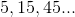Which is the greater quantity?

(a) The seventh term of the sequence

(b)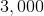(a) is greater

(a) and (b) are equal

It is impossible to tell from the information given

(b) is greater

(a) is greater

Explanation:

The common ratio of the sequence is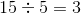, so the next four terms of the sequence are: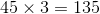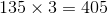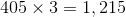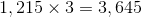, the seventh term, which is greater than 3,000.

### Example Question #1 : Sets

Seven students are running for student council. A student can vote for any three. Steve wants to vote for his girlfriend Marsha.

Which is the greater quantity?

(a) The number of ways Steve can fill out his ballot so that he can vote for Marsha

(b)(b) is greater

It is impossible to tell from the information given

(a) and (b) are equal

(a) is greater

(a) and (b) are equal

Explanation:

Since one of Steve's votes has already been decided, this is a choice of two out of the remaining six without regard to order - that is, the number of combinations of two out of a set of six. This is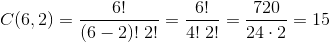### Example Question #1 : How To Find The Missing Part Of A List

Eight students are running for student council. A student can vote for any two. Phil only wants to vote for male candidates; Sandee only wants to vote for female candidates.

Which is the greater quantity?

(a) The number of ways Phil can fill out his ballot so that he can vote for two male candidates

(b) The number of ways Sandee can fill out her ballot so that she can vote for two female candidates

(b) is greater

It is impossible to tell from the information given

(a) is greater

(a) and (b) are equal

It is impossible to tell from the information given

Explanation:

This question is impossible to answer, because it is not known how many candidates are male and how many are female.

### Example Question #1 : Sets

An arithmetic sequence begins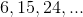Which is the greater quantity?

(a) The fiftieth term of the sequence

(b)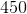(a) and (b) are equal

(b) is greater

It is impossible to tell from the information given

(a) is greater

(b) is greater

Explanation:

The common difference of the sequence is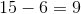, so the fiftieth term will be: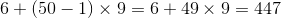which is less than 450.

### Example Question #3 : How To Find The Missing Part Of A List

An arithmetic sequence begins: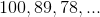Which is the greater quantity?

(a)(b) The tenth term of the sequence

(a) and (b) are equal

It is impossible to tell from the information given

(b) is greater

(a) is greater

(b) is greater

Explanation:

The common difference of the sequence is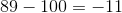. The tenth term of the sequence is.

This makes (b) greater.

### Example Question #3 : How To Find The Missing Part Of A List

The Fibonacci sequence begins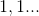with each subsequent term being the sum of the previous two.

Which is the greater quantity?

(a) The product of the eighth and tenth terms of the Fibonacci sequence

(b) The square of the ninth term of the Fibonacci sequence

(a) is greater

(b) is greater

(a) and (b) are equal

It is impossible to tell from the information given

(b) is greater

Explanation:

By beginning with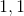and taking the sum of the previous two terms to get each successive term, we can generate the Fibonacci sequence: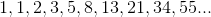(a) The eighth and tenth terms are 21 and 55; their product is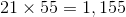(b) The ninth term is 34; its square is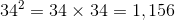.

(b) is greater

### Example Question #171 : Data Analysis And Probability

The Fibonacci sequence beginswith each subsequent term being the sum of the previous two.

Which is the greater quantity?

(a) The product of the fifth and eighth terms of the Fibonacci sequence

(b) The product of the sixth and seventh terms of the Fibonacci sequence

(a) and (b) are equal

It is impossible to tell from the information given

(a) is greater

(b) is greater

(a) is greater

Explanation:

By beginning withand taking the sum of the previous two terms to get each successive term, we can generate the Fibonacci sequence: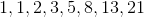(a) The fifth and eighth terms are 5 and 21; their product is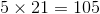(b) The sixth and seventh terms are 8 and 13; their product is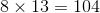(a) is greater.

### Example Question #171 : Data Analysis And Probability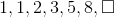What is the next number in the series above?Explanation:

First, you must determine the pattern in the series. The pattern is adding the two preceding terms to give you the next term (ex: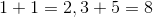). Therefore, apply that rule to calculate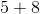, which gives you 13 as the next term.

### Example Question #171 : Data Analysis And Probability

Five candidates are running for school board. A voter chooses two of the candidates.

Which is the greater quantity?

(a) The number of ways a voter can mark his or her ballot

(b)(b) is greater

It is impossible to tell from the information given

(a) and (b) are equal

(a) is greater

(b) is greater

Explanation:

This is a choice of two out of five without regard to order - that is, the number of combinations of two out of a set of five. This is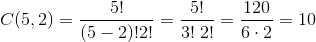There are ten possible ways to choose, and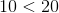.

### Example Question #391 : Isee Middle Level (Grades 7 8) Quantitative Reasoning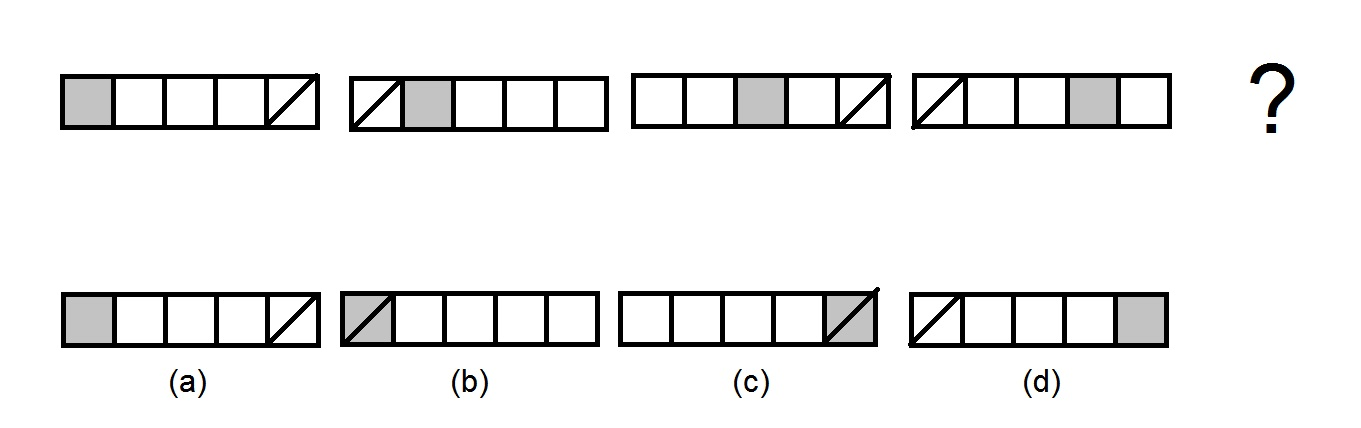Refer to the above diagram. The top row gives a sequence of figures. Which figure on the bottom row comes next?

Figure (a)

Figure (b)

Figure (d)

Figure (c)